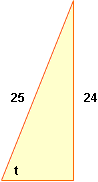Quandaries and Queries name: Weisu secondary school student I have some questions about pre-calculus. (1) (2(cos(x))^2)+3sin(x)-1=0 (2) sin(x)cos(x)=(1/2) (3) 3sin(x)=1+cos(2x) (4) tan(x)*csc(x)=csc(x)+1 (5) sin(arccsc(8/5)) (6) tan(arcsin(24/25)) (7) arccos(cos(11pi/6)) the last problem uses radian measure. I tried the problems, and here is what I have so far: (1) (cos(x))^2=1-(sin(x))^2, so substituting, 2(1-sin(x)^2)+3sin(x)-1=0. Distributing, 2-(2sin(x)^2)+3sin(x)-1=0. From here, I do not know where to go. (2) I don't know where to start here. (3) I don't know where to start here. (4) starting with the original equation, tan(x)*csc(x) =csc(x)+1, divide both sides by csc(x): tan(x)=1-sin(x). From here, I don't know what to do. (5) I don't know where to start here. (6) I don't know where to start here. (7) I don't know where to start here. Weisu Hi Weisu, I can help with a few of your problems. You have so far 2-(2sin(x)2)+3sin(x)-1=0, that is -2sin(x)2+3sin(x)+1=0. This is a quadratic in sin(x). If you write y=sin(x) then this becomes -2y2+3y+1=0. This doesn't factor (are you sure that you have the original problem written down correctly) but you can solve it with the general quadratic If you multiply both sides by 2 you get 2sin(x)cos(x)=1. But 2sin(x)cos(x)=sin(2x) so the problem becomes sin(2x)=1. Expand cos(2x) in terms of sin(x) and this problem will aslo be a quadratic in sin(x) like problem 1. For problems 5, 6 and 7 draw a right triangle and write down what you know. For example for problem 6.In the diagram sin(t) = 24/25, so tan(arcsin(24/25)) is the tangent of t. Use Pythagoras' Theorem to find the length of the third side of the triangle, and then find tan(t). Penny Go to Math Central